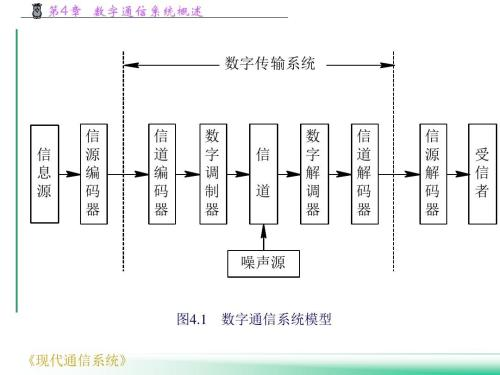27 篇文章 5 订阅

通信原理教程chapter1

消息,信息和信号

1. 假设你家里有矿,形形色色的矿
2. 有一天你闲得无聊,去看别人挖矿

信息量

1. 假设你家的矿坍塌了,现在采的矿石都是金银铜铁混合的.
2. 出现金银铜铁的概率是独立的,不可能互相转化
显然:
P 金 &lt; P 银 &lt; P 铜 &lt; P 铁 P_{金} &lt; P_{银} &lt; P_{铜} &lt; P_{铁}

I = − l o g a 1 P ( x ) = l o g a P ( x ) I = - log_{a}\frac1{P(x)} = log_a P(x)

I [ P ( x 1 ) P ( x 2 ) … … ] = I [ P ( x 1 ) ] + I [ P ( x 2 ) ] + … … I[P(x_1)P(x_2)……]=I[P(x_1)]+I[P(x_2)]+……

1. 如果a=2 , 则信息量的单位为比特(bit),简称为b (最为常用)
2. 如果a=e , 则信息量的单位为奈特(nat)
3. 如果a=10,则信息量的单位为哈特莱(hartley)

P 金 = P 银 = P 铜 = P 铁 P_{金} = P_{银} = P_{铜} = P_{铁}

I = l o g 2 1 1 / M = l o g 2 M I=log_2 \frac1{1/M} = log_2 M

数字通信系统

通信系统基本概念

模拟通信系统

1. 系统传输质量的准则:信号噪声功率比(信噪比)
2. 基本问题:连续波形的参量估值问题

数字通信系统

1. 系统传输质量的准则:产生错误判决的概率(误码率)
2. 理论基础:统计判决理论

数字通信的优点:

1. 由于有中继站对失真信号的整形,所以到接收端依然有高质量的接收信号.
2. 可以采用纠错编码等差错控制技术,提高系统抗干扰能力.(汉明码)
3. 可以采用数字加密技术,提高系统的保密性
4. 可以综合传输各种模拟和数字输入消息,便于存储和处理
5. 设计和制造更容易,体积更小,重量更轻
6. 可以通过信源编码进行压缩,提高信道利用率
7. 输出信噪比随带宽按指数规律增长.

数字通信系统模型数字通信系统的主要性能指标

传输速率

1. 码元速率( R B R_B ):单位时间(s)内传输的码元数目,单位是"波特(Band)"
2. 信息速率( R b R_b ):单位时间(s)内传输的信息量,单位是"比特/秒(b/s)"
对于一个系统而言,我们是没有办法确知下一个信号是什么从而评判系统的性能指标的,所以这里引入一个平均信息量
假设离散信息的种类数目为N,第i个消息出现的概率为 p i p_i ,则有:
I = ∑ i = 1 N p i l o g 2 ( 1 / p i ) ( b ) I=\sum^N_{i=1}p_i log_2 (1/p_i) (b)
其实概念很简单,就是对各个消息根据概率做加权平均数而已…

I = l o g 2 N I=log_2 N

3条便可,相当于识别了3次,相当于数据结构的二分查找法.

I N = l o g 2 8 = 3 I_N = log_2 8 = 3

R b = R B l o g 2 M R_b =R_B log_2 M

1. 消息速率( R M R_M )单位时间内传输的消息数目.

错误率

1. 误码率( P e P_e ):
P e = 错 误 接 收 码 元 数 目 / 传 输 码 元 总 数 目 P_e = 错误接收码元数目/传输码元总数目
2. 误比特率( P b P_b ):
P b = 错 误 接 收 比 特 数 目 / 传 输 比 特 总 数 目 P_b = 错误接收比特数目/传输比特总数目
3. 误字率( P w P_w ):
P b = 错 误 接 收 字 数 目 / 传 输 字 总 数 目 P_b = 错误接收字数目/传输字总数目
特别地,如果一个字由k比特组成:
P w = 1 − ( 1 − P e ) k P_w = 1-(1-P_e)^k

信道

电磁场四道大题

1已知电场求磁场
1. 已知电场求磁场
这里有两种情况:
1. 均匀平面波
2. 不知道哪里来的随意电磁波
分成这两个的主要原因是,我们都知道求TEM波的电磁场转换是有一个简便方式的(P137)
H ⃗ ( r ⃗ ) = 1 η a ⃗ n X E ⃗ ( r ⃗ ) = 1 η ( a ⃗ n X E ⃗ 0 + ) e − j k n ∙ r \vec H(\vec r)=\frac1{\eta}\vec a_n X \vec E(\vec r) = \frac1{\eta} (\vec a_n X \vec E^+_0) e^{-jk_n\bullet r}
η = μ ε \eta=\sqrt{\frac \mu\varepsilon}
这个公式虽然非常好用,但是一定要注意他的细节:
3. 必须是TEM波
4. 注意方向的转变

∇ × E ⃗ = j ω μ H ⃗ \nabla \times \vec E=j\omega \mu \vec H

2平行传输线,已知阻抗求传输线长度
1. 一定要先去看看我的电磁学乱七八糟的符号(五),大概弄懂各个参量的定义和意义
2. 至于用阻抗求长度的话,要明确一个叫做波长的概念:
λ = 2 π β \lambda = \frac{2\pi}{\beta}
3. 还有一个叫做阻抗转换的概念:
第一种情况是我前面博客所写的 λ 4 \frac \lambda 4 阻抗变换性,下面的公式,这个时候你就要把相关反射系数的概念给弄清楚.
第二种情况就是书上的例题(P207)还有一点点的课后习题
看着公式觉得难的时候就写写课后习题吧,应用起来还是蛮简单的
3理想导体平面,垂直极化斜入射
1. 一定要先去看看我的电磁学乱七八糟的符号(三)
2. …其实不知道写什么,毕竟我的博客里面偏偏就有这种情况的分析
4时变磁场,在滑轨上连电阻,求电流
1. 这是一条,高中奥数题
2. 公式在我的电磁学乱七八糟的符号(一),里面的感应电动势,如果电阻已知的话,其实就是求电压
3. B和S其实定其中一个,变另外一个那还好说,直接套公式就求出来了.
4. 最怕的是B和S同时变化,这种情况下就要用定义式
ε i n ≜ − d ψ d t \varepsilon_{in} \triangleq -\frac{d \psi}{d t}
其中 ψ \psi 为磁通量
里面的 ψ \psi 在变化的时候,微分变量分解会有两个东西:
d ψ = B ⃗ d S ⃗ d t + S ⃗ d B ⃗ d t d\psi = \vec B \frac{d \vec S}{d t} + \vec S \frac{d \vec B}{d t}
再求就完事了.

结语

09-19869

10-30
07-13
05-06
11-30
01-07
04-10
12-241209
11-11771
12-041443
07-092万+
03-172万+hhhhorrible

¥2 ¥4 ¥6 ¥10 ¥20余额支付 (余额：-- )扫码支付获取中扫码支付点击重新获取扫码支付1.余额是钱包充值的虚拟货币，按照1:1的比例进行支付金额的抵扣。
2.余额无法直接购买下载，可以购买VIP、C币套餐、付费专栏及课程。余额充值